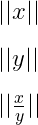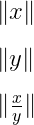# How to write norm symbol in LaTeX like ||a||?

There are many ways to represent the norm symbol in a latex document. However, in all cases, the shape of this symbol does not increase or decrease dynamically.

So, in this tutorial, we will discuss the best method which will be the best practice for you.

 Symbol Norm symbol Type Mathematics Package Default, physics, mathtools Commands \|..\|, \lVert..\rVert, \Vert Example \lVert x \rVert → ||x||

Most of the time you will use the double bar on both sides of the expression or variable to represent the norm symbol that is on your keyboard.

\documentclass{article}
\begin{document}
$|| x ||$
$|| x^{2} ||$
$|| \frac{x}{y} ||$
\end{document}

Output :You can use a backslash single bar instead of a double bar, which will return you the same output. For example

\documentclass{article}
\begin{document}
$\| x \|$
$\| y \|$
$\| \frac{x}{y} \|$
\end{document}

Output :You can use \lVert and \rVert or \Vert commands for double bar symbols. However, for each command, the letter V will be capitalized. Because latex is case-sensitive.

\documentclass{article}
\usepackage{physics}
\begin{document}
$\Vert x \Vert$
% -- Use physics package for \lVert and \rVert commands --
$\lVert y \rVert$
$\lVert \frac{x}{y} \rVert$
\end{document}

Output :When you use this symbol multiple times in a document, it may not feel good to write such a large syntax over and over again. So, in this case, the big syntax is converted into a small command with the help of \newcommand.

\documentclass{article}
\usepackage{amsmath}
\newcommand\norm{\lVert#1\rVert}
\newcommand\normx{\Vert#1\Vert}
\begin{document}
$\norm{x }$
$\normx{y}$
$\normx{\frac{x}{y}}$
\end{document}

Output :## Big or responsive size norm symbol

In all the commands discussed above for the norm symbol, the shape of the symbol does not increase and decrease dynamically according to the shape of the expression.

\documentclass{article}
\usepackage{amsmath}
\newcommand\norm{\lVert#1\rVert}
\newcommand\normx{\Vert#1\Vert}
\begin{document}
$\norm{\frac{x}{y}}$
$\norm{\sum_{i=1}^{n}x_{i}}$
$\normx{\frac{w}{v}}$
$\normx{w}$
\end{document}

Output :However, for this responsive size norm symbol, you need to use the \left and \right commands before the present command. for example

\documentclass{article}
\usepackage{amsmath}
\newcommand\norm{\left\lVert#1\right\rVert}
\newcommand\normx{\left\Vert#1\right\Vert}
\begin{document}
$\norm{\frac{x}{y}}$
$\norm{\sum_{i=1}^{n}x_{i}}$
$\normx{\frac{w}{v}}$
$\normx{w}$
\end{document}

Output :## Use physics package for norm symbol in LaTeX

The physics package contains the predefine \norm command for this symbol. And this command will return you the norm symbol of responsive size.

\documentclass{article}
\usepackage{physics}
\begin{document}
$\norm{\frac{x}{y}}$
$\norm{A_{i,j}}$
$\norm{\frac{w}{v}}$
$\norm{\sum_{i=1}^{n}\frac{x_i}{\abs{x}}}$
\end{document}

Output :Second, you can use four types of big commands for different sizes with the norm command.

\documentclass{article}
\usepackage{physics}
\begin{document}
$\norm\big{\frac{v}{w}} \; \norm\Big{\frac{v}{w}} \; \norm\bigg{\frac{v}{w}} \; \norm\Bigg{\frac{v}{w}}$
\end{document}

Output :And if you want a norm symbol of constant size, use the * sign with the command like \norm*.

\documentclass{article}
\usepackage{physics}
\begin{document}
$\norm*{w}$
$\norm*{\frac{v}{w}}$
$\norm*{V_{i,j}}$
$\norm*{\sum_{i=1}^{n}x_{i}}$
\end{document}

Output :## Use mathtools package for norm symbol in LaTeX

There are no predefined commands in mathtools packages like physics package for this symbol, you can define new commands with the help of \DeclarePairedDelimiter.

\documentclass{article}
\usepackage{mathtools}
\DeclarePairedDelimiter\norm{\lVert}{\rVert}
\begin{document}
$\norm{w}$
$\norm{\frac{v}{w}}$
$\norm{\sum_{i=1}^{n}(x_{i})^2}$
$\sum_{i=1}^{n}\frac{w}{\norm{w_i}}$
\end{document}

Output :You notice the output above, the size of the norm symbol is not adjusting with the size of the expression. For this, you need to use the star symbol along with the norm command.

\documentclass{article}
\usepackage{mathtools}
\DeclarePairedDelimiter\norm{\lVert}{\rVert}
\begin{document}
$\norm*{w}$
$\norm*{\frac{v}{w}}$
$\norm*{\sum_{i=1}^{n}(x_{i})^2}$
$\sum_{i=1}^{n}\frac{w}{\norm*{w_i}}$
\end{document}

Output :And you can use four types of big commands like \big, \Big, \bigg, and \Bigg as optional arguments with this command. Which returns you a variety of sizes. For example

\documentclass{article}
\usepackage{mathtools}
\DeclarePairedDelimiter\norm{\lVert}{\rVert}
\begin{document}
$\norm[\big]{\frac{x}{y}} \; \norm[\Big]{\frac{x}{y}} \; \norm[\bigg]{\frac{x}{y}} \; \norm[\Bigg]{\frac{x}{y}}$
\end{document}

Output :## Norm of vector in LaTeX

You will notice that the norm symbol is used with the vector. For example

\documentclass{article}
\usepackage{physics,amsmath}
\begin{document}
$\norm{k\vec{a}}=\abs{k}\norm{\vec{a}}$
$\vu*{a}=\frac{\vec{a}}{\norm{\vec{a}}}$
$\norm{\vec{u}} = \sqrt{u^2_1 + u^2_2 + \cdots + u^2_n}$
\end{document}

Output :In latex, the best practice is to use the physics package for the norm symbol. Because there are predefined commands in the physics package, you don’t need to write large syntax separately.

And there are a lot of examples in this tutorial to help you understand. I hope you have found the answer to your question.#### Md Jidan Mondal

LaTeX expert with over 10 years of experience in document preparation and typesetting. Specializes in creating professional documents, reports, and presentations using LaTeX.